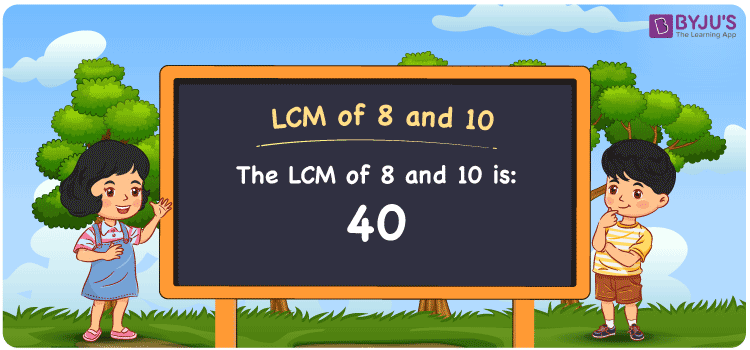Defend yourself better by mastering the science of immunity and vaccines. Secure your free spot, now! Defend yourself better by mastering the science of immunity and vaccines. Secure your free spot, now!

# LCM of 8 and 10

LCM of 8 and 10 is 40. LCM represents the least common factor or multiple of any two or more given integers. Students can learn the procedure of finding the LCM and HCF of given numbers by referring to HCF and LCM anytime for free. We can calculate the LCM of given numbers by using the prime factorisation, division method and listing multiples. Learn the technique of finding the least common multiple of 8 and 10 in simple language in this article.

## What is LCM of 8 and 10?

The answer to this question is 40.## How to Find LCM of 8 and 10?

We can find the LCM of 8 and 10 by using the three methods shown below:

• Prime Factorisation
• Division method
• Listing the multiples

### LCM of 8 and 10 Using Prime Factorisation Method

By prime factorisation method, we can write 8 and 10 as the product of prime numbers, such that;

8 = 2 × 2 × 2

10 = 2 × 5

LCM (8, 10) = 2 × 2 × 2 × 5 = 40

### LCM of 8 and 10 Using Division Method

In this method, we divide the numbers 8 and 10 by their prime numbers to calculate their LCM. The product of these divisors depicts the least common multiple of 8 and 10. This can be done as follows.

 2 8 10 2 4 5 2 2 5 5 1 5 x 1 1

No more further division can be done.

Hence, LCM (8, 10) = 2 × 2 × 2 × 5 = 40

### LCM of 8 and 10 Using Listing the Multiples

By listing the multiples of 8 and 10, we can determine their least common multiple with ease. The below table shows the multiples of 8 and 10.

 Multiples of 8 Multiples of 10 8 10 16 20 24 30 32 40 40 50 48 60

LCM (8, 10) = 40

## Related Articles

LCM calculator

Least Common Multiple

LCM of Two Numbers

Prime Factorization and Division Method for LCM and HCF

LCM Formula

LCM with Examples

## Video Lesson on Applications of LCM## Solved Examples

Q. 1: The GCD and LCM of two numbers are 2 and 40. If one of the numbers is 10. Calculate the other number.

Solution: Let the other number be z

GCD × LCM = 10 × z

z = (GCD × LCM)/10

z = (2 × 40)/10

z = 8

Therefore the other number is 8.

Q. 2: What is the LCM if the product of two numbers is 80 and their GCD is 2?

Solution: Given,

Product of numbers = 80

GCD = 2

LCM × GCD = Product of numbers

LCM = Product of numbers / GCD

LCM = 80/2

LCM = 40

Thus the LCM is 40.

## Frequently Asked Questions on LCM of 8 and 10

Q1

### What is the LCM of 8 and 10?

The LCM of 8 and 10 is 40.
Q2

### 42 is the LCM of 8 and 10. True or False.

False. 40 is the LCM of 8 and 10.
Q3

### How do you find the LCM of 8 and 10 by Prime Factorization?

Prime factors of 8 = 2 × 2 × 2

Prime factors of 10 = 2 × 5

LCM = 2 × 2 × 2 × 5 = 40